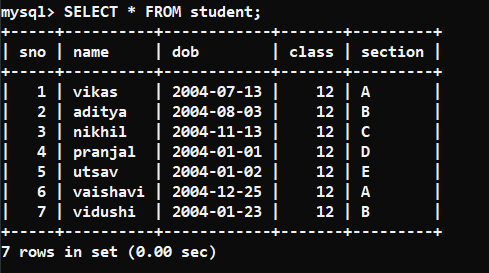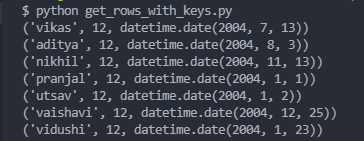Open in App
Not now

# How to get all rows with keys provided in a list using SQLalchemy?

• Last Updated : 28 Feb, 2022

In this article, we are going to get all the rows with keys provided in a list using SQLAlchemy.

Database used:Note: For this post, we are going to get “name“, “class” and “dob” keys from the student table.

## Installation:

Syntax to install SQLAlchemy:

`pip install sqlalchemy pymysql`

Note: pymysql is a dependency that we need to install for this post

## Stepwise Implementation

### Step 1 :

The first step includes importing the module and connecting to the database. You can do that by the following code:

## Python3

 `from` `sqlalchemy ``import` `create_engine ` ` `  `user , password , host , database ``=` `'root'` `, ``'123'` `, ``'localhost'` `, ``'geeksforgeeks'` `engine ``=` `create_engine(url``=``f``'mysql+pymysql://{user}:{password}@{host}/{database}?charset=utf8'``) ` ` `  `connection ``=` `engine.connect() `

### Step 2 :

In the second step, we will define the table name and all the keys inside their respective variables.

## Python3

 `table_name ``=` `'student'` `keys ``=` `[``'name'``,``'class'``,``'dob'``] `

### Step 3 :

Now, firstly in SQL to get particular keys, you need to specify them in the query.

The syntax of the SQL query is:

`SELECT key1 , key2 , ..... keyN FROM table_name;`

So, we will form a query in python. Firstly we will use the “join” method of python to join all the keys (separated by a comma) and then using “f-string” we will form a query following the above syntax.

So our python code for that will be:

## Python3

 `keys_joined ``=` `","``.join(keys) ` `query ``=` `f``'SELECT {keys_joined} FROM {table_name}'`

### Step 4 :

Now, finally, we will execute the query and print out all the elements in the fetched result using a for loop.

## Python3

 `result ``=` `connection.execute(query) ` `for` `elem ``in` `result: ` `    ``print``(elem)`

### Example:

In this example, we are combining all the above-mentioned step into a single code and then displaying all rows with keys provided in a list.

## Python3

 `from` `sqlalchemy ``import` `create_engine ` ` `  `user , password , host , database ``=` `'root'` `, ``'123'` `, ``'localhost'` `, ``'geeksforgeeks'` `engine ``=` `create_engine(url``=``f``'mysql+pymysql://{user}:{password}@{host}/{database}?charset=utf8'``) ` ` `  `connection ``=` `engine.connect() ` ` `  `table_name ``=` `'student'` `keys ``=` `[``'name'``,``'class'``,``'dob'``] ` ` `  `keys_joined ``=` `","``.join(keys) ` `query ``=` `f``'SELECT {keys_joined} FROM {table_name}'` ` `  `result ``=` `connection.execute(query) ` `for` `elem ``in` `result: ` `    ``print``(elem) `

Output:My Personal Notes arrow_drop_up
Related Articles